Name
• String
• Substring
• Search
Edit
The Boyer-Moore algorithm is considered as the most efficient string-matching algorithm in usual applications. A simplified version of it or the entire algorithm is often implemented in text editors for the «search» and «substitute» commands.

The algorithm scans the characters of the pattern from right to left beginning with the rightmost one. In case of a mismatch (or a complete match of the whole pattern) it uses two precomputed functions to shift the window to the right. These two shift functions are called the good-suffix shift (also called matching shift and the bad-character shift (also called the occurrence shift).

Assume that a mismatch occurs between the character x[i]=a of the pattern and the character y[i+j]=b of the text during an attempt at position j.
Then, x[i+1 .. m-1]=y[i+j+1 .. j+m-1]=u and x[i]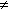y[i+j]. The good-suffix shift consists in aligning the segment y[i+j+1 .. j+m-1]=x[i+1 .. m-1] with its rightmost occurrence in x that is preceded by a character different from x[i] (see figure 13.1).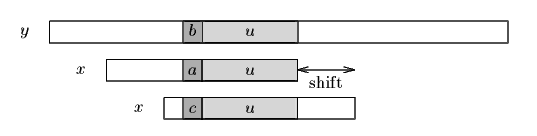Figure 13.1. The good-suffix shift, u re-occurs preceded by a character c different from a.
If there exists no such segment, the shift consists in aligning the longest suffix v of y[i+j+1 .. j+m-1] with a matching prefix of x (see figure 13.2).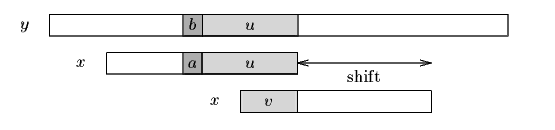Figure 13.2. The good-suffix shift, only a suffix of u re-occurs in x.

The bad-character shift consists in aligning the text character y[i+j] with its rightmost occurrence in x[0 .. m-2]. (see figure 13.3)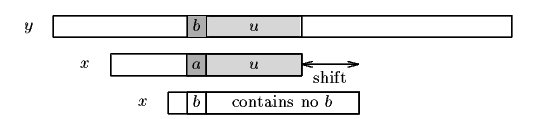Figure 13.3. The bad-character shift, a occurs in x.

If y[i+j] does not occur in the pattern x, no occurrence of x in y can include y[i+j], and the left end of the window is aligned with the character immediately after y[i+j], namely y[i+j+1] (see figure 13.4).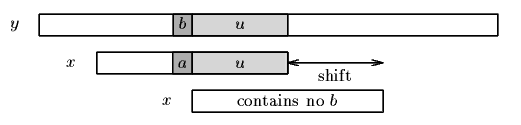Figure 13.4. The bad-character shift, b does not occur in x.

Note that the bad-character shift can be negative, thus for shifting the window, the Boyer-Moore algorithm applies the maximum between the the good-suffix shift and bad-character shift. More formally the two shift functions are defined as follows.

The good-suffix shift function is stored in a table bmGs of size m+1.
Let us define two conditions:
• Cs(i, s): for each k such that i < k < m, s  k or x[k-s]=x[k] and
• Co(i, s): if s <i then x[i-s]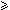x[i]

Then, for 0  i < m: bmGs[i+1]=min{s>0 : Cs(i, s) and Co(i, s) hold}
and we define bmGs as the length of the period of x. The computation of the table bmGs use a table suff defined as follows: for 1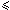i < m, suff[i]=max{k : x[i-k+1 .. i]=x[m-k .. m-1]}

The bad-character shift function is stored in a table bmBc of size . For c in : bmBc[c] = min{i : 1i <m-1 and x[m-1-i]=c} if c occurs in x, m otherwise.

Tables bmBc and bmGs can be precomputed in time O(m+) before the searching phase and require an extra-space in O(m+). The searching phase time complexity is quadratic but at most 3n text character comparisons are performed when searching for a non periodic pattern. On large alphabets (relatively to the length of the pattern) the algorithm is extremely fast. When searching for am-1b in bn the algorithm makes only O(n / m) comparisons, which is the absolute minimum for any string-matching algorithm in the model where the pattern only is preprocessed.
C